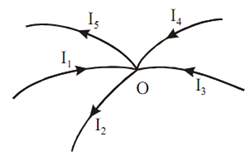## Kirchhoffs Current Law Assignment Help

Assignment Help: >> Kirchhoffs Laws - Kirchhoffs Current Law

Kirchhoff's Current Law (KCL):

Kirchhoff's current law states that algebraic sum of all the current meeting at a point or junction is zero. As this law relates the current passing through the circuit, this is known as Kirchhoff's Current Law (KCL).

In Figure , applying KCL to the junction, we have

I1  + I3  + I4  - I2  - I5  = 0Figure: KCL at Junction

∑ Current entering the junction = ∑ Current leaving the junction.

KCL also mention the law of conservation of charges and may also be states as at any node of a circuit, at every instant of time, the sum of incoming currents is equal to the sum of outgoing currents.